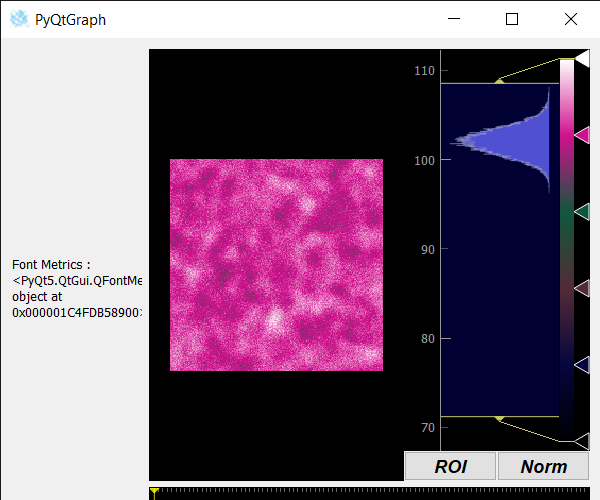# PyQtGraph – Getting Font Metrics of the Image View

• Last Updated : 26 May, 2022

This article discusses how can we get font metrics of the image view object in PyQTGraph. In metal typesetting, a font has a particular size, weight and style of a typeface. Each font has a matched set of type and a typeface consisting of a range of fonts that shares an overall design. It can be set with the help of setFont method. Font metrics are the measurements of characters in a particular font, which allow you to evenly space and uniformly align lines of text.

PyQtGraph is a graphics and user interface Python library for functionalities commonly required in designing and science applications. Its provides fast, interactive graphics for displaying data (plots, video, etc.). Implements many features like displaying 2D and 3D image data. For 3D data, a z-axis slider is displayed allowing the user to select which frame will be displayed. Displays histogram of image data with movable region defining the dark/light levels and editable gradient provides a color lookup table for reference.

For creating an image view ImageView() is used

Syntax:

imv = pg.ImageView()

To get font metrics of the image view, fontMetrics() is used. It takes no arguments and returns a QFontMetrics object.

Syntax :

imv.fontMetrics()

Implementation:

## Python3

 `# importing Qt widgets``from` `PyQt5.QtWidgets ``import` `*` `# importing system``import` `sys` `# importing numpy as np``import` `numpy as np` `# importing pyqtgraph as pg``import` `pyqtgraph as pg``from` `PyQt5.QtGui ``import` `*``from` `PyQt5.QtCore ``import` `*`  `# Image View class``class` `ImageView(pg.ImageView):` `    ``# constructor which inherit original``    ``# ImageView``    ``def` `__init__(``self``, ``*``args, ``*``*``kwargs):``        ``pg.ImageView.__init__(``self``, ``*``args, ``*``*``kwargs)` `class` `Window(QMainWindow):` `    ``def` `__init__(``self``):``        ``super``().__init__()` `        ``# setting title``        ``self``.setWindowTitle(``"PyQtGraph"``)` `        ``# setting geometry``        ``self``.setGeometry(``100``, ``100``, ``600``, ``500``)`  `        ``# icon``        ``icon ``=` `QIcon(``"skin.png"``)` `        ``# setting icon to the window``        ``self``.setWindowIcon(icon)` `        ``# calling method``        ``self``.UiComponents()` `        ``# showing all the widgets``        ``self``.show()` `        ``# setting fixed size of window``        ``#self.setFixedSize(QSize(600, 500))` `    ``# method for components``    ``def` `UiComponents(``self``):` `        ``# creating a widget object``        ``widget ``=` `QWidget()` `        ``# creating a label``        ``label ``=` `QLabel(``"Geeksforgeeks Image View"``)` `        ``# setting minimum width``        ``label.setMinimumWidth(``130``)` `        ``# making label do word wrap``        ``label.setWordWrap(``True``)` `        ``# setting configuration options``        ``pg.setConfigOptions(antialias``=``True``)` `        ``# creating image view  object``        ``imv ``=` `ImageView()` `        ``# Create random 3D data set with noisy signals``        ``img ``=` `pg.gaussianFilter(np.random.normal(size``=``(``200``, ``200``)), (``5``, ``5``)) ``*` `20` `+` `100` `        ``# setting new axis to image``        ``img ``=` `img[np.newaxis, :, :]` `        ``# decay data``        ``decay ``=` `np.exp(``-``np.linspace(``0``, ``0.3``, ``100``))[:, np.newaxis, np.newaxis]` `        ``# random data``        ``data ``=` `np.random.normal(size``=``(``100``, ``200``, ``200``))``        ``data ``+``=` `img ``*` `decay``        ``data ``+``=` `2` `        ``# adding time-varying signal``        ``sig ``=` `np.zeros(data.shape[``0``])``        ``sig[``30``:] ``+``=` `np.exp(``-``np.linspace(``1``, ``10``, ``70``))``        ``sig[``40``:] ``+``=` `np.exp(``-``np.linspace(``1``, ``10``, ``60``))``        ``sig[``70``:] ``+``=` `np.exp(``-``np.linspace(``1``, ``10``, ``30``))` `        ``sig ``=` `sig[:, np.newaxis, np.newaxis] ``*` `3``        ``data[:, ``50``:``60``, ``30``:``40``] ``+``=` `sig` `        ``# setting image to image view``        ``# Displaying the data and assign each frame a time value from 1.0 to 3.0``        ``imv.setImage(data, xvals``=``np.linspace(``1.``, ``3.``, data.shape[``0``]))` `        ``## Set a custom color map``        ``colors ``=` `[``            ``(``0``, ``0``, ``0``),``            ``(``4``, ``5``, ``61``),``            ``(``84``, ``42``, ``55``),``            ``(``15``, ``87``, ``60``),``            ``(``208``, ``17``, ``141``),``            ``(``255``, ``255``, ``255``)``        ``]` `        ``# color map``        ``cmap ``=` `pg.ColorMap(pos``=``np.linspace(``0.0``, ``1.0``, ``6``), color``=``colors)` `        ``# setting color map to the image view``        ``imv.setColorMap(cmap)` `        ``# Creating a grid layout``        ``layout ``=` `QGridLayout()` `        ``# minimum width value of the label``        ``label.setFixedWidth(``130``)` `        ``# setting this layout to the widget``        ``widget.setLayout(layout)` `        ``# adding label in the layout``        ``layout.addWidget(label, ``1``, ``0``)` `        ``# plot window goes on right side, spanning 3 rows``        ``layout.addWidget(imv, ``0``, ``1``, ``3``, ``1``)` `        ``# setting this widget as central widget of the main window``        ``self``.setCentralWidget(widget)` `        ``# creating a font object``        ``font ``=` `QFont(``'Arial'``, ``11``)` `        ``# making font italic and bold``        ``font.setItalic(``True``)``        ``font.setBold(``True``)` `        ``# setting font to the image view``        ``imv.setFont(font)` `        ``# getting font metrics of the image view``        ``value ``=` `imv.fontMetrics()` `        ``# setting text to the label``        ``label.setText(``"Font Metrics : "` `+` `str``(value))`   `# create pyqt5 app``App ``=` `QApplication(sys.argv)` `# create the instance of our Window``window ``=` `Window()` `# start the app``sys.exit(App.``exec``())`

Output :My Personal Notes arrow_drop_up